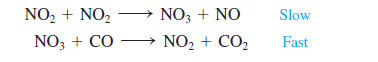# Problem: The mechanism for the gas-phase reaction of nitrogen dioxide with carbon monoxide to form nitric oxide and carbon dioxide is thought to be Write the rate law expected for this mechanism. What is the overall balanced equation for the reaction?

###### FREE Expert Solution

When a reaction mechanism is given, the rate law can be determined using the slow step in the reaction mechanism.

rate law only involves the reactants
▪ the coefficients of the reactants in the slow step are the order of the reaction with respect to that reactant
▪ Rate Law has a general form of:

98% (341 ratings)###### Problem Details

The mechanism for the gas-phase reaction of nitrogen dioxide with carbon monoxide to form nitric oxide and carbon dioxide is thought to beWrite the rate law expected for this mechanism. What is the overall balanced equation for the reaction?

Frequently Asked Questions

What scientific concept do you need to know in order to solve this problem?

Our tutors have indicated that to solve this problem you will need to apply the Reaction Mechanism concept. If you need more Reaction Mechanism practice, you can also practice Reaction Mechanism practice problems.

What professor is this problem relevant for?

Based on our data, we think this problem is relevant for Professor Collins' class at TAMU.

What textbook is this problem found in?

Our data indicates that this problem or a close variation was asked in Chemistry: An Atoms First Approach - Zumdahl Atoms 1st 2nd Edition. You can also practice Chemistry: An Atoms First Approach - Zumdahl Atoms 1st 2nd Edition practice problems.